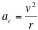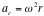# Centripetal Acceleration

Yesterday in class we saw how we need to define what type of speed we are talking about, linear speed vs angular speed.  We looked at LEGO gear ratios and what happens when we mix gears on the same axle.  The rotational speed is the same, but the linear speed is different.  And what happens when we join gears on separate axles; the linear speed is the same but the rotational speeds are different.  We can calculate the gear ratios by counting teeth (or reading the LEGO piece which tells us how many teeth are on it).

We left class considering two examples:  The conical pendulum was our first example.  Here, a pendulum is kept in motion by a propeller (or flapping wings).  The pig/owl/bat was traveling in a circle.  We explored various methods for trying to determine the angular speed of the flying animal.

In the second example, your physics teacher defied gravity by swinging a bucket of water over his head.  Leonardo thought I had glued the water in, but as we saw, there was no glue involved.  Leonardo was so inspired that he decided he would try the experiment himself with some LEGO pieces.  He wants to try it with water too.  I think we’ll wait until summer and practice outside.

Today in class we explored this idea we call centripetal force.  In truth, the is no such thing as centripetal force, just as there is no such thing as up force, down force, left force, right force.  Centripetal is a direction which means “towards the center.”  We define a force, which is in a centripetal direction as one which will cause an object to move in a circle.  We could study other curves (such as elliptical motion, parabolic, or any number of curves) but for simplicity we limit our study to circular motion.

Examples of this include:

Tension in a mass on the end of a string

Planets circling the sun due to the force of gravity

Electrons orbiting an atom due to the Coulomb (electric) force

Particles circling in the Large Hadron Collider due to the Lorentz (magnetic) force

When we first introduced acceleration we defined it as a change in velocity.  This could be a change in speed or a change in the direction of that velocity.  As I mentioned, we could define this change in direction for any amount of curvature, but the equations for a perfect circle will be easiest to work with at this level.  Most physics texts will present a relationship between this centripetal acceleration for an object moving in a circle with a constant radius, r, at a constant speed, vI never liked this equation as it is counter-intuitive.  It does make sense to me that the centripetal acceleration increases with speed.  But acceleration is NOT inversely proportional to the radius of curvature.  It is better to use angular speed, ω to explain centripetal acceleration and express this asWe can make this substitution because remember that v = rω

There is an illusion known as centrifugal force.  This is merely your own inertia acting against you.  Just as when you step on the gas in a car you feel a mysterious force pushing you back into your seat, the same sensation can be felt in the turns.  Your inertia wants to keep going in a straight line.  So the centrifugal force is this sensation of wanting to continue outwards in a straight line tangent to the radius of the circle.